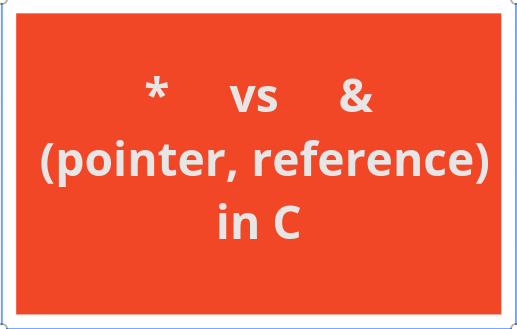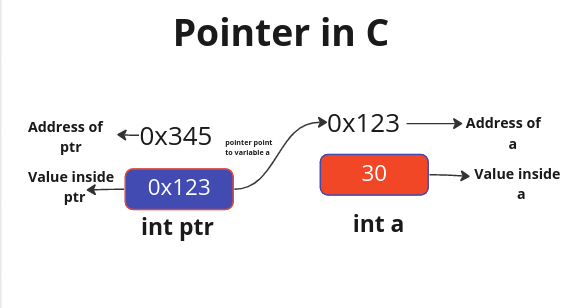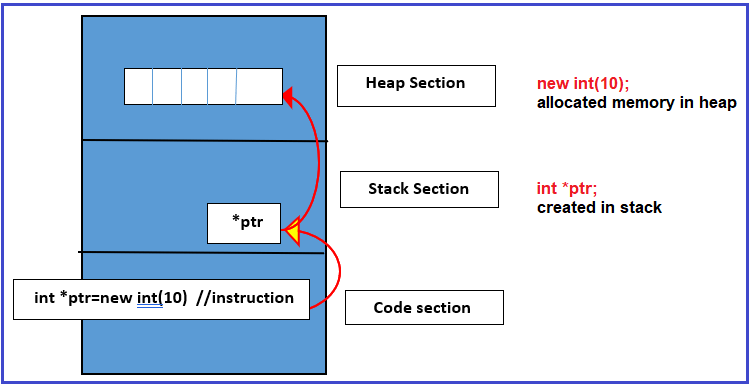×

Search anything:

# * vs & (pointer, reference) in C

#### C++Get this book -> Problems on Array: For Interviews and Competitive ProgrammingC programming language is a strong statically typed language. This means that we are responsible for the declaration of variable type, dynamic memory allocation and free of such memory space after it has been used explicitly. Pointer and reference are very important concepts in C.

In this article, we will look at the concept of pointer and reference in C.

1. Introduction to pointer and reference
2. Types of pointer
3. Application of pointer and reference
4. Example of pointer and reference

### Introduction to pointer and referenceUnlike ordinary variables which store value for easy retrieving of such value for later use, pointer is a special type of variable. It is a variable that stores the address of another variable.
Every variable is stored and takes up specific space in the memory. The space taken depends on the type of data stored in it. The address of a variable is a figure representing where the variable sits or occupies in the memory. It is a territory of such variables. It is usually denoted by a hexadecimal value (0X54d42fa6). Asterisk (*) symbol is used to declare pointer variables.

We've established that a pointer stores the address of a variable. Then, how can we have access to the address of the variable? This is where the concept of reference comes in. The ampersand (&) symbol is used to get access to the address of a variable stored in a pointer. Let's take a quick example to explain all that we've said.

``````  // The syntax for a C pointer
ptr = &variable
``````
``````   // example of a pointer declaration and assignment
int a = 10;
int *ptr;
ptr = &a;
``````

In the first line of the code, we declared an integer variable a and assigned 10 as its value. On the next line, declared an integer pointer or a pointer to an integer using the (*) symbol. This means that it can only store addresses of integer variables. Then, we proceed to assign the address of the variable a to the ptr* by using the (&) symbol

### Types of pointers.

In C, there are four (4) major types of pointers. Which are:

1. Null pointer
2. Void Pointer
3. Wild Pointer
4. Dangling Pointer

#### Null Pointer

A null pointer is a pointer that points to null values. We create a null pointer by assigning a null value to a pointer at the time of declaration. It is useful to prevent a dangling pointer.

``````     int *ptr = NULL;
``````

#### Void Pointer

A void pointer is a type of pointer that does not have a specific data type. It can store the address of any variable type and it can be typecast to any data type. It is also called a generic pointer. void keyword is used to declare a void pointer

``````     void *ptr = NULL;
``````

#### Wild Pointer

A wild pointer is also called an uninitialized pointer. It is a type of pointer that points to any arbitrary memory location. The wild pointer should be avoided as it can cause unexpected results in our program.

``````     void *ptr;
``````

#### Dangling Pointer

A dangling pointer occurs when the objects that it pointed to get deallocated, freed or out of scope. To prevent a dangling pointer, the pointer should be assigned to null after the memory space it points to gets destroyed.

``````    int *ptr = malloc(sizeof(int) * 5);
free(ptr);
ptr // Dangling pointer

ptr = NULL; // prevents the pointer from dangling
``````

### Application of pointer and reference

There are different use cases for pointers and references. In this article at OpenGenus, we will briefly discuss about the common use cases:

1. Initialization of a string literal
In C, string is not part of the primitive data type. It is only considered as a sequential of characters or array of characters that ends with null terminating character ('\0'). There are different ways of declaring a string data type in c. One of them is through string literal.

``````    char *str = "This is a string literal!";
``````

String literal is another way of initializing strings with the help of a pointer. It points to the first character in the string.

2. Pass by reference
Arguments can be passed to a function either by value or by reference depending on the choice of outcome.

Pass by value means passing a variable to a function using the variable name.
When a variable is passed into a function, a local copy of the variable is created in the called function and any update made on it would not affect the original copy in the calling function.

``````    int x = 10;
int y = 20;

// Pass by value
swap(x, y)

// Value of x and y remain the same after swapping
printf("%d, %d\n", x, y); // 10, 20
``````

Pass by reference means passing the address of variable to a function. If your intention is for the changes made on the variables passed into a function reflected in the original copy, then you should pass by reference. When a variable is passed by reference, no duplicate of the variable is created rather it is pointing directly to the original copy and any changes made would reflect in the original copy.

The below example demonstrates how to pass by value and by reference:

``````    int x = 10;
int y = 20;

// Pass by reference
swap(&x, &y);

// Value of x and y were succesfully swapped
printf("%d, %d\n", x, y); // 20, 10
``````

3. Dereferencing
Asterisk (*) symbol is used to declare a pointer and also used to dereference pointer. Dereference is a way of accessing the actual value stored in the variable referenced by a pointer. It is used to get access to the value and also to manipulate the value.
This might sound confusing but you will understand it better with a few examples.

``````    int x = 10;
int *ptr = &x;

printf("%p\n", ptr); // 0x1f3546772

// Access the value stores in the address ptr is pointing to
printf("%d, %d\n", x, *ptr); // 10, 10

// Update the value stores in ptr to 20
*ptr = 20;

printf("%d, %d\n", x, *ptr); // 20, 20

*ptr == x // true
``````

3. Dynamic memory allocationDynamic memory allocation happens in the heap segment of the memory. Due to the nature of the execution context and stack section of memory, having access to the local variable of a called function after it has finished executing is impossible. To make it possible, we will need to dynamically allocate memory for the local variable. Functions such as malloc(), calloc(), realloc() and free() are used for dynamic memory allocation. When one of the first three is used, the address of the memory space is returned.

This is an example of a program that dynamically allocates 100 bytes of memory in the heap.

``````    char *ptr;
ptr = malloc(sizeof(char) * 100);
``````

*ptr points to the first character of the 100 characters in the heap, which can be utilised to get access to the rest of the characters using pointer arithmetic or indexing. It is crucial to note that, it is our responsibility to free or deallocate any memory space allocated in the heap after we are done using it.

``````    free(ptr);
ptr = NULL; // To prevent dangling pointer
``````

### Example of pointer and reference

Solving some problems requires swap operation either on two integers, two characters or any data type at all to get it done. To make the swapping persistent, the concept of pointer and reference need to be considered.
In this example, we are going to write a simple program that swaps two integers using the concept of pointer and reference.

``````    void swap(int *x, int *y)
{
int temp = *x;
*x = *y;
*y = temp;
}

int main(void)
{
int x = 10;
int y = 20;

// Before swapping
printf("%d, %d\n", x, y); // 10, 20

// Pass by reference
swap(&x, &y);

// After swapping
printf("%d, %d\n", x, y); // 20, 10

return (0);
}
``````

x and y are declared and assigned 10 and 20 respectively. We tried to print the value of x and y before swapping the two integers.

Address of &x and &y are passed to the swap function. As we know that only the pointer variable can store the address of another variable, that is why the prototype of the swap function takes a pointer to *x and a pointer to *y.

Inside the swap function, we declare a temp variable to the temporary store value of x by dereferencing it. Afterwards, the two integers are swapped and the control returns to the calling function which is the main function. By this time, the value of x has successfully been swapped with the value of y as shown after printing.

It is pertinent to note that, the changes made to both variables wouldn't have reflected, if we had tried to swap the variables by values instead of reference. The time complexity of swap function is O(1).